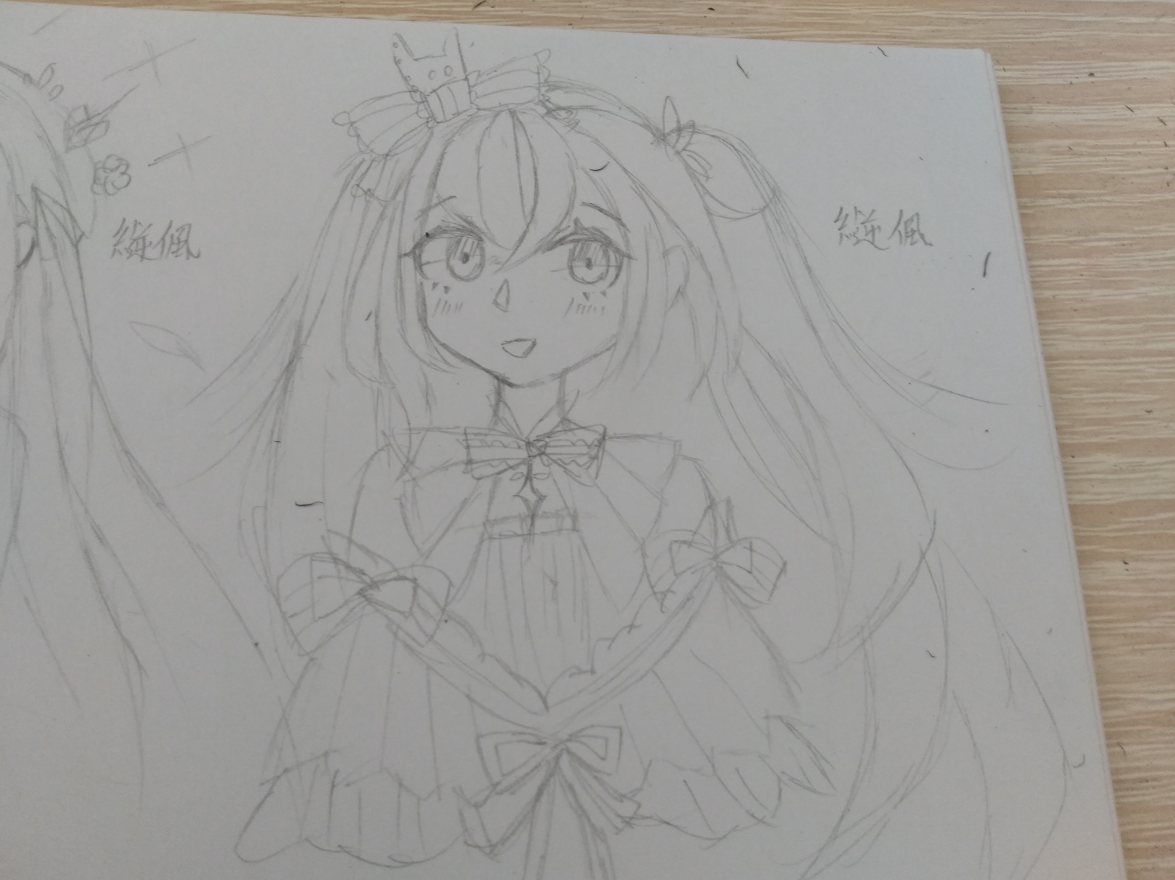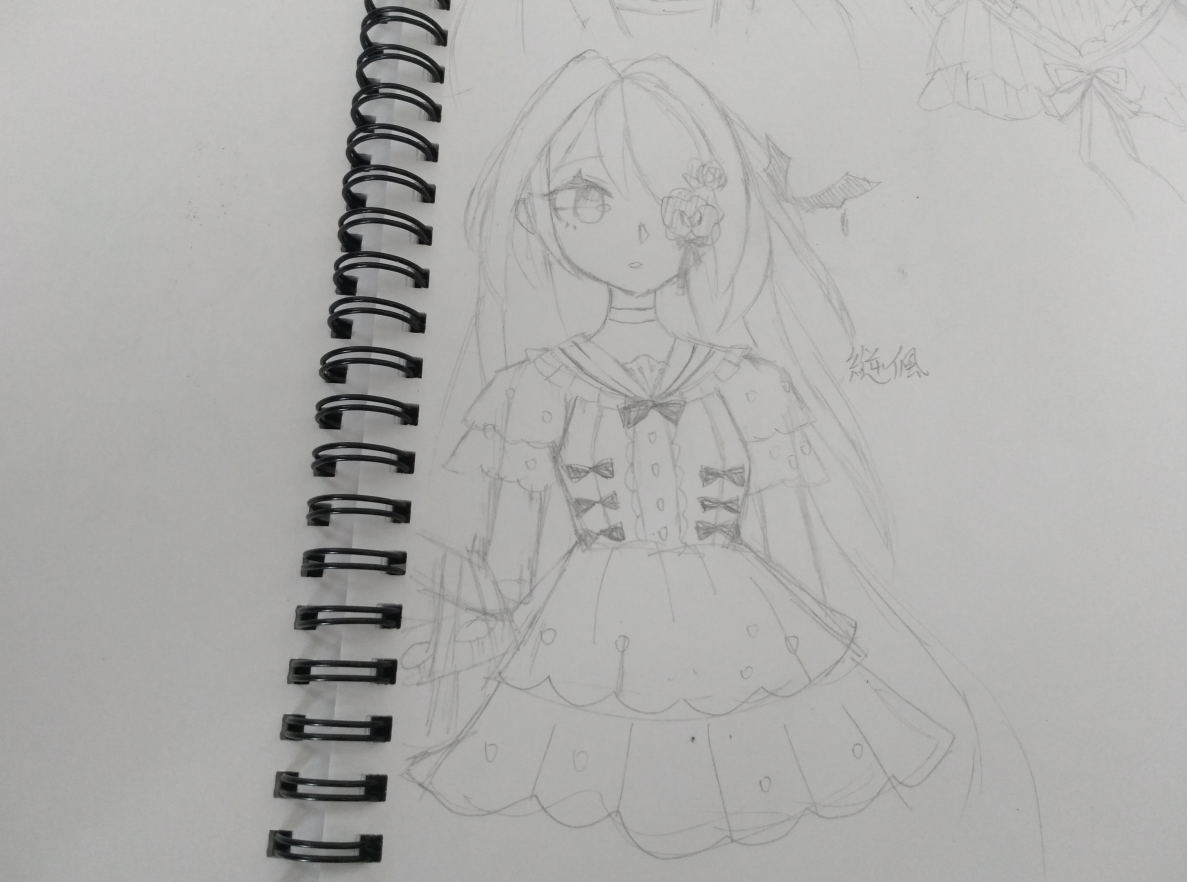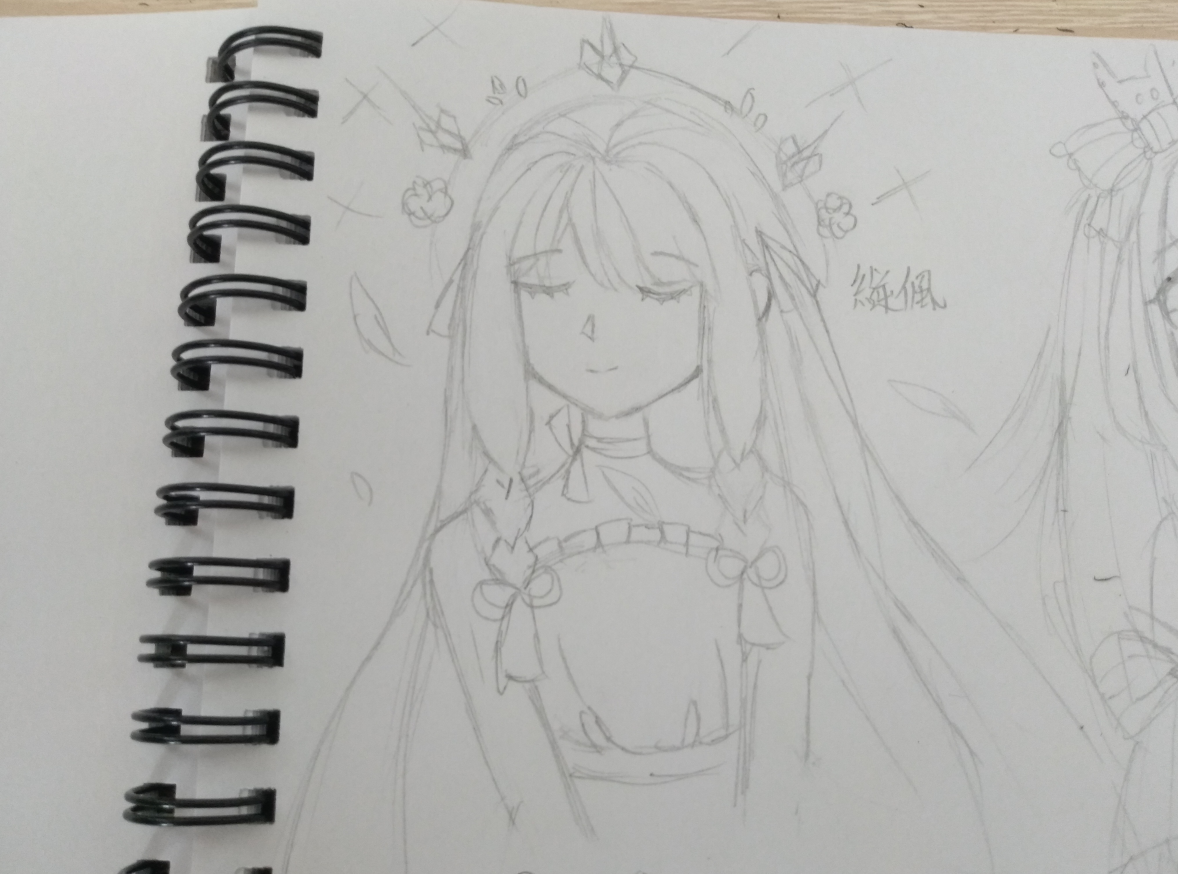<<返回奥雅之光圈 回应/阅读：75/1168

# 【縌偑】放假了想接点形象卡！（新画风+极粗糙警告Lv17 聆风遥.

4渐入佳境=w=w=w=w=w=w=w=w=w=w=w=w=w=w=w=w=w=w=Lv19 煮鱼是帅哥

5小有名气Lv17 执言

7自成一派

ddd康康我！！！

“幸得识卿桃花面，自此阡陌多暖春。”Lv17 聆风遥.

4渐入佳境

（这个是参赛作品↓）

=w=w=w=w=w=w=w=w=w=w=w=w=w=w=w=w=w=w=Lv7 神姬奈Liz

1圈子菜鸟

ddLv17 聆风遥.

4渐入佳境

20L的

=w=w=w=w=w=w=w=w=w=w=w=w=w=w=w=w=w=w=Lv23 华子小小

5小有名气Lv15 江綄吟

3略有小成

ddd，神仙画画，敲好看！吹爆

<<返回奥雅之光圈 回应/阅读：75/1168
+1

## 热门专题

+......Emotional.